Courses

# Antiderivatives - Integration, Business Mathematics & Statistics B Com Notes | EduRev

## B Com : Antiderivatives - Integration, Business Mathematics & Statistics B Com Notes | EduRev

The document Antiderivatives - Integration, Business Mathematics & Statistics B Com Notes | EduRev is a part of the B Com Course Business Mathematics and Statistics.
All you need of B Com at this link: B Com

#### Antiderivatives An antiderivative of a function f is a function whose derivative is f . In other words, F is an antiderivative of f if F' = f . To find an antiderivative for a function f , we can often reverse the process of differentiation. For example, if f = x4, then an antiderivative of f is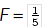which can be found by reversing the power rule. Notice that not only is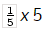an antiderivative of f , but so are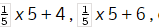etc. In fact, adding or subtracting any constant would be acceptable.

This should make sense algebraically, since the process of taking the derivative (i.e. going from F to f ) eliminates the constant term of F.

Because a single continuous function has infinitely many antiderivatives, we do not refer to "the antiderivative", but rather, a "family" of antiderivatives, each of which differs by a constant. So, if Fis an antiderivative of f , then G = F + c is also an antiderivative of f , and F and G are in the same family of antiderivatives.

#### Indefinite Integral

The notation used to refer to antiderivatives is the indefinite integral.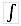f (x)dx means the antiderivative of f with respect to x . If F is an antiderivative of f , we can writef (x)dx = F + c . In this context, c is called the constant of integration.

1. x n dx =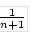x n+1 + c as long as n does not equal -1. This is essentially the power rule for derivatives in reverse
2.cf (x)dx = cf (x)dx . That is, a scalar can be pulled out of the integral.
3.(f (x) + g(x))dx =f (x)dx +g(x)dx . The antiderivative of a sum is the sum of the antiderivatives.
4.sin(x)dx = - cos(x) + ccos(x)dx = sin(x) + csec2(x)dx = tan(x) + c
These are the opposite of the trigonometric derivatives.
Offer running on EduRev: Apply code STAYHOME200 to get INR 200 off on our premium plan EduRev Infinity!

122 videos|142 docs

,

,

,

,

,

,

,

,

,

,

,

,

,

,

,

,

,

,

,

,

,

,

,

,

;